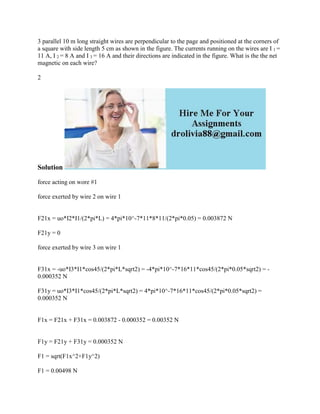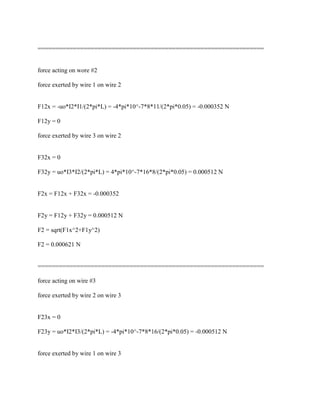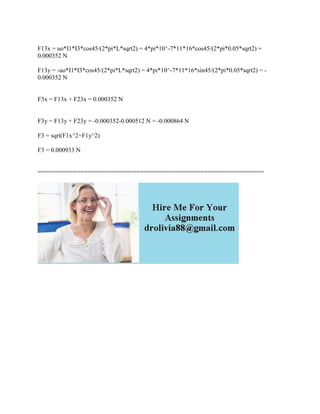Diese Präsentation wurde erfolgreich gemeldet.

# 3 parallel 10 m long straight wires are perpendicular to the page and.docx

Anzeige
Anzeige
Anzeige
Anzeige
Anzeige
Anzeige
Anzeige
Anzeige
Anzeige
Anzeige
Anzeige×

1 von 3 Anzeige

# 3 parallel 10 m long straight wires are perpendicular to the page and.docx

3 parallel 10 m long straight wires are perpendicular to the page and positioned at the corners of a square with side length 5 cm as shown in the figure. The currents running on the wires are I 1 = 11 A, I 2 = 8 A and I 3 = 16 A and their directions are indicated in the figure. What is the the net magnetic on each wire?
2
Solution
force acting on wore #1
force exerted by wire 2 on wire 1
F21x = uo*I2*I1/(2*pi*L) = 4*pi*10^-7*11*8*11/(2*pi*0.05) = 0.003872 N
F21y = 0
force exerted by wire 3 on wire 1
F31x = -uo*I3*I1*cos45/(2*pi*L*sqrt2) = -4*pi*10^-7*16*11*cos45/(2*pi*0.05*sqrt2) = -0.000352 N
F31y = uo*I3*I1*cos45/(2*pi*L*sqrt2) = 4*pi*10^-7*16*11*cos45/(2*pi*0.05*sqrt2) = 0.000352 N
F1x = F21x + F31x = 0.003872 - 0.000352 = 0.00352 N
F1y = F21y + F31y = 0.000352 N
F1 = sqrt(F1x^2+F1y^2)
F1 = 0.00498 N
================================================================
force acting on wore #2
force exerted by wire 1 on wire 2
F12x = -uo*I2*I1/(2*pi*L) = -4*pi*10^-7*8*11/(2*pi*0.05) = -0.000352 N
F12y = 0
force exerted by wire 3 on wire 2
F32x = 0
F32y = uo*I3*I2/(2*pi*L) = 4*pi*10^-7*16*8/(2*pi*0.05) = 0.000512 N
F2x = F12x + F32x = -0.000352
F2y = F12y + F32y = 0.000512 N
F2 = sqrt(F1x^2+F1y^2)
F2 = 0.000621 N
================================================================
force acting on wire #3
force exerted by wire 2 on wire 3
F23x = 0
F23y = uo*I2*I3/(2*pi*L) = -4*pi*10^-7*8*16/(2*pi*0.05) = -0.000512 N
force exerted by wire 1 on wire 3
F13x = uo*I1*I3*cos45/(2*pi*L*sqrt2) = 4*pi*10^-7*11*16*cos45/(2*pi*0.05*sqrt2) = 0.000352 N
F13y = -uo*I1*I3*cos45/(2*pi*L*sqrt2) = 4*pi*10^-7*11*16*sin45/(2*pi*0.05*sqrt2) = -0.000352 N
F3x = F13x + F23x = 0.000352 N
F3y = F13y + F23y = -0.000352-0.000512 N = -0.000864 N
F3 = sqrt(F1x^2+F1y^2)
F3 = 0.000933 N
================================================================
.

3 parallel 10 m long straight wires are perpendicular to the page and positioned at the corners of a square with side length 5 cm as shown in the figure. The currents running on the wires are I 1 = 11 A, I 2 = 8 A and I 3 = 16 A and their directions are indicated in the figure. What is the the net magnetic on each wire?
2
Solution
force acting on wore #1
force exerted by wire 2 on wire 1
F21x = uo*I2*I1/(2*pi*L) = 4*pi*10^-7*11*8*11/(2*pi*0.05) = 0.003872 N
F21y = 0
force exerted by wire 3 on wire 1
F31x = -uo*I3*I1*cos45/(2*pi*L*sqrt2) = -4*pi*10^-7*16*11*cos45/(2*pi*0.05*sqrt2) = -0.000352 N
F31y = uo*I3*I1*cos45/(2*pi*L*sqrt2) = 4*pi*10^-7*16*11*cos45/(2*pi*0.05*sqrt2) = 0.000352 N
F1x = F21x + F31x = 0.003872 - 0.000352 = 0.00352 N
F1y = F21y + F31y = 0.000352 N
F1 = sqrt(F1x^2+F1y^2)
F1 = 0.00498 N
================================================================
force acting on wore #2
force exerted by wire 1 on wire 2
F12x = -uo*I2*I1/(2*pi*L) = -4*pi*10^-7*8*11/(2*pi*0.05) = -0.000352 N
F12y = 0
force exerted by wire 3 on wire 2
F32x = 0
F32y = uo*I3*I2/(2*pi*L) = 4*pi*10^-7*16*8/(2*pi*0.05) = 0.000512 N
F2x = F12x + F32x = -0.000352
F2y = F12y + F32y = 0.000512 N
F2 = sqrt(F1x^2+F1y^2)
F2 = 0.000621 N
================================================================
force acting on wire #3
force exerted by wire 2 on wire 3
F23x = 0
F23y = uo*I2*I3/(2*pi*L) = -4*pi*10^-7*8*16/(2*pi*0.05) = -0.000512 N
force exerted by wire 1 on wire 3
F13x = uo*I1*I3*cos45/(2*pi*L*sqrt2) = 4*pi*10^-7*11*16*cos45/(2*pi*0.05*sqrt2) = 0.000352 N
F13y = -uo*I1*I3*cos45/(2*pi*L*sqrt2) = 4*pi*10^-7*11*16*sin45/(2*pi*0.05*sqrt2) = -0.000352 N
F3x = F13x + F23x = 0.000352 N
F3y = F13y + F23y = -0.000352-0.000512 N = -0.000864 N
F3 = sqrt(F1x^2+F1y^2)
F3 = 0.000933 N
================================================================
.

Anzeige
Anzeige

### 3 parallel 10 m long straight wires are perpendicular to the page and.docx

1. 1. 3 parallel 10 m long straight wires are perpendicular to the page and positioned at the corners of a square with side length 5 cm as shown in the figure. The currents running on the wires are I 1 = 11 A, I 2 = 8 A and I 3 = 16 A and their directions are indicated in the figure. What is the the net magnetic on each wire? 2 Solution force acting on wore #1 force exerted by wire 2 on wire 1 F21x = uo*I2*I1/(2*pi*L) = 4*pi*10^-7*11*8*11/(2*pi*0.05) = 0.003872 N F21y = 0 force exerted by wire 3 on wire 1 F31x = -uo*I3*I1*cos45/(2*pi*L*sqrt2) = -4*pi*10^-7*16*11*cos45/(2*pi*0.05*sqrt2) = - 0.000352 N F31y = uo*I3*I1*cos45/(2*pi*L*sqrt2) = 4*pi*10^-7*16*11*cos45/(2*pi*0.05*sqrt2) = 0.000352 N F1x = F21x + F31x = 0.003872 - 0.000352 = 0.00352 N F1y = F21y + F31y = 0.000352 N F1 = sqrt(F1x^2+F1y^2) F1 = 0.00498 N
2. 2. ================================================================ force acting on wore #2 force exerted by wire 1 on wire 2 F12x = -uo*I2*I1/(2*pi*L) = -4*pi*10^-7*8*11/(2*pi*0.05) = -0.000352 N F12y = 0 force exerted by wire 3 on wire 2 F32x = 0 F32y = uo*I3*I2/(2*pi*L) = 4*pi*10^-7*16*8/(2*pi*0.05) = 0.000512 N F2x = F12x + F32x = -0.000352 F2y = F12y + F32y = 0.000512 N F2 = sqrt(F1x^2+F1y^2) F2 = 0.000621 N ================================================================ force acting on wire #3 force exerted by wire 2 on wire 3 F23x = 0 F23y = uo*I2*I3/(2*pi*L) = -4*pi*10^-7*8*16/(2*pi*0.05) = -0.000512 N force exerted by wire 1 on wire 3
3. 3. F13x = uo*I1*I3*cos45/(2*pi*L*sqrt2) = 4*pi*10^-7*11*16*cos45/(2*pi*0.05*sqrt2) = 0.000352 N F13y = -uo*I1*I3*cos45/(2*pi*L*sqrt2) = 4*pi*10^-7*11*16*sin45/(2*pi*0.05*sqrt2) = - 0.000352 N F3x = F13x + F23x = 0.000352 N F3y = F13y + F23y = -0.000352-0.000512 N = -0.000864 N F3 = sqrt(F1x^2+F1y^2) F3 = 0.000933 N ================================================================